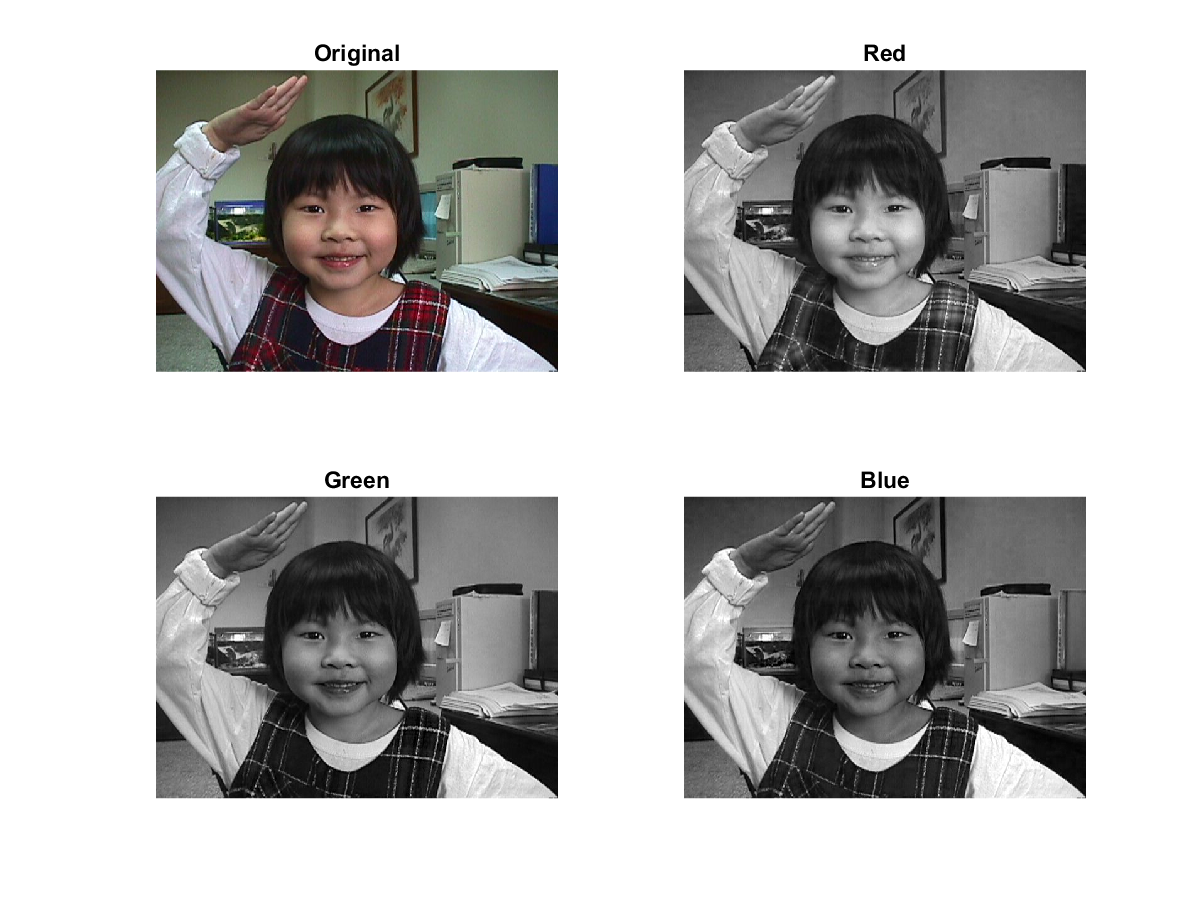Part 1

1. Create a 3D array: 假設資訊系及電機系在下列各年度的人口統計下：

資訊系：
 類別 大一新生 學士畢業生 碩士畢業生 博士畢業生 年份 2001 98 94 80 5 2002 105 97 87 6 2003 121 110 89 8

電機系：
 類別 大一新生 學士畢業生 碩士畢業生 博士畢業生 年份 2001 99 98 85 10 2002 113 101 87 12 2003 120 115 80 15

請寫一個簡短的程式getMdArray.m來建立一個3維矩陣A，以表示上述資料。

2. Computing over a 3D array: 請寫一個程式 mdStatistics.m 來由上題的矩陣 A 計算出下列各數值：
1. 資訊系在 2001、2002 和2003 年之間的每年平均新生、學士畢業生、碩士畢業生及博士畢業生的個數。
2. 資訊系和電機系在各個年度的新生總數。
3. 3 年來電機系和資訊系共畢業多少位碩士生？
4. 3 年來電機系和資訊系共有多少畢業生？
5. 在哪一年，電機系和資訊系合計有最多的碩士畢業生？
6. 在哪一年，電機系和資訊系的學士畢業生差額最大？
7. 在哪幾年，電機系收的新生數目比資訊系多？
8. 資訊系三年來每年的學士畢業生對大一新生的比例平均值為何？

Part 2

1. Matrix dimensions: Suppose A is a 5x4x3 array. Give the dimensions of the results after the following operations:
1. sum(A)
2. sum(A, 1)
3. sum(A, 2)
4. sum(A, 3)
5. sum(A(:))
6. sum(sum(sum(A)))
7. A(:, :)
8. A(:)
9. sum(A(:, :))
10. sum(A(:, :), 2)
2. Conversion between linear indexing and multi-dimensional indexing:
1. Suppose that A is a 2-dimensional array of size $m \times n$. Given A(i, j), how to find the linear index y such that A(y) = A(i, j)? Given A(y), how to find i and j such that A(i, j)=A(y)?
2. Suppose that A is a 3-dimensional array of size $p \times q \times r$. Given A(i, j, k), how to find the linear index y such that A(y) = A(i, j, k)? Given A(y), how to find i, j and k such that A(i, j, k)=A(y)?
3. How do you generalize the above formulas to deal with a n-dimensional array of size $u_1 \times u_2 \times \cdots \times u_n$?
3. Matrix concatenation: Suppose that A = [1 2; 3 4] and B = [1 0; 0 1]. What is returned by each of the following statements?
1. cat(1, A, B)
2. cat(2, A, B)
3. cat(3, A, B)
4. cat(4, A, B)
4. Sum of ND arrays: An array Z can be created by the following statements: A = [1 1 1 1; 2 2 2 2; 3 3 3 3]; B = [0 0 0 0; 1 1 1 1; 1 2 3 4]; Z = cat(3, A, B); What are the sizes of the returned arrays of the following statements?
1. sum(Z, 1)
2. sum(Z, 2)
3. sum(Z, 3)
4. sum(Z, 4)
5. Reorganize a 3D array: A true-color image (such as a jpg file) of size mxn can be represented as a 3-dimensional array of size mxnx3, where each layer (or page) is the pixel intensity of R (red), G (green), and B (blue), respectively. For instance, you can read the following image file and display the size of A together with its R, G, and B components:Example 1: 11-多維陣列/rgbDisplay01.mA=imread('annie19980405.jpg'); fprintf('Dim of A = %s\n', mat2str(size(A))); subplot(221); imshow(A); title('Original'); subplot(222); imshow(A(:, :, 1)); title('Red'); subplot(223); imshow(A(:, :, 2)); title('Green'); subplot(224); imshow(A(:, :, 3)); title('Blue'); Dim of A = [480 640 3]For certain application of image processing, you need to reshape A into a 2-dimensional matrix B of size 3x(mxn), where each column is the RGB intensity vector of a pixel. (For instance, the first column of B is the RGB intensity of pixel (1,1), the second column is the RGB intensity of pixel (2,1), and so on.) Please write a function myReshape.m that can achieve this goal, with the following I/O format:
B=myReshape(A);

Note that you cannot use any loop or iteration in your program. Instead, you need to construct B by a smart way of indexing A in a vectorized manner.

Hint:

You can use the command "reshape" to transform matrix A directly, or you can create an index matrix first. If A is a 2x3x3 matrix, then the index matrix which can be used for creating matrix B is shown next. $$\left[ \begin{matrix} 1 & 2 & 3 & 4 & 5 & 6\\ 7 & 8 & 9 & 10 & 11 & 12\\ 13 & 14 & 15 & 16 & 17 & 18\\ \end{matrix} \right]$$

MATLAB程式設計：入門篇